# DAV Class 7 Maths Chapter 10 Brain Teasers Solutions

The DAV Class 7 Maths Solution and DAV Class 7 Maths Chapter 10 Brain Teasers Solutions of Construction of Triangles offer comprehensive answers to textbook questions.

## DAV Class 7 Maths Ch 10 Brain Teasers Solutions

Question 1.
A. Tick (✓) the correct option:
(i) A closed figure in a plane having three sides and three angles is known as —
(a) square
(b) rectangle
(c) triangle
(d) pentagon
(c) triangle

A closed figure in a plane having three sides and three angles is called a triangle.
Hence, (c) is the correct option.

(ii) What are the possible dimensions for constructing a triangle of perimeter of 5 m?
(a) 1m, 1m, 3m
(b) 1m, 2m, 2m
(c) 5m, 1.5m, 3m
(d) 1m, 1.5m, 2.5m
(b) 1m, 2m, 2m

The data 1 m, 2 m, 2 m satisfy the triangle inequality property because (1 + 2 > 2, 2 + 2 > 1, 1 + 2 > 2) and, therefore, it is possible to construct a triangle of perimeter 5 m.
Hence, (b) is the correct option.Question 2.
Construct a ∆ABC in which ∠A = 65°, ∠B = 75° and BC = 4 cm.
Steps of Construction: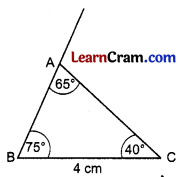Draw BC = 4 cm Draw ∠B = 75°
Draw ∠C = 40°
[∵∠C = 180° – (∠A + ∠B)
= 180° – (75° + 65°)
= 180° – 140° = 40°]
The two angle lines meet at A.
Triangle ABC is the required triangle.

Question 3.
Construct a ∆ABC in which ∠C = 90°
Steps of Construction: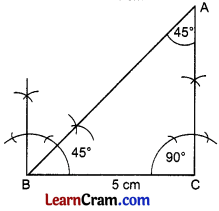1. Draw BC = 5 cm
2. Draw ∠C = 90°
3. Draw ∠B = 45°
[∵ ∠B = 90° – ∠A = 90° – 45° = 45°]
4. The two angle lines meet at A.
5. ∆ABC is a required right triangle.

Question 4.
Construct a ∆ABC in which AB = 4cm, AC = 5.2 cm and ∠A = 135°. Also bisect ∠A and ∠B.
Steps of Construction: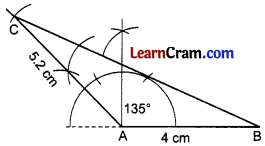1. Draw AB = 4 cm
2. Draw ∠A= 135°
3. Draw AC = 5.2 cm A 4 Cm
4. Join BC.
5. ∆ABC is a required triangle.

Question 5.
Construct a ∆ABC in which AB = 6 cm, BC = 4.5 cm and AC = 5.9 cm. Also construct perpendicular bisectors of sides AC and AB.
Steps of Construction: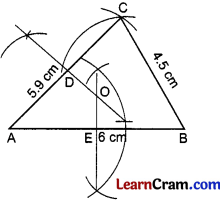1. Draw AB = 6 cm
2. Draw two arcs with centre A and B and of radius 5.9 cm and 4.5 cm respectively to meet at C.
3. Join AC and BC.
4. ∆ABC is the required triangle.
5. Draw the perpendicular bisectors of AB and AC at E and D respectively to meet at O.Question 6.
Draw a triangle ABC, with AB = 2 cm, BC = 4 cm and CA = 3 cm. Draw a line through A, perpendicular to line BC.
Steps of Construction:1. Draw BC = 4 cm
2. Draw two arcs with centre B and C and with radius 2 cm and 3 cm respectively to meet at A.
3. Join AB and AC.
4. ∆ABC is the required triangle.
5. Draw an arc with centre A such that it cuts BC at F and G.
6. Draw two arcs with centre F and G to cut each other at E.
7. Join AE to meet BC at D.
8. AD is the required perpendicular from A to BC.

Question 7.
Construct a right angled triangle in which sides containing the right angle are 3 cm and 4 cm. Measure the hypotenuse.
Steps of Construction:1. Draw AB = 4 cm
2. Draw ∠B = 90° and cut BC = 3 cm
3. Join AC.
4. ∆ABC is the required right triangle.
5. AC = 5 cm.

Question 8.
Construct a right ∆ABC such that BC = 4 cm and the hypotenuse makes an angle of 30° at B. Verify that AB = 2AC.
Steps of Construction:1. Draw BC = 4 cm
2. Draw ∠C = 90° and ∠B = 30°
3. The two angle lines meet each other at A.
4. ∆ABC is the required right triangle.
5. AC = 2.3 cm and AB = 4.6 cm
∴ AB = 2AC Hence Verified.

## DAV Class 7 Maths Chapter 10 HOTS

Question 1.
On a line segment AB = 4 cm, construct a square.
Let us first draw a rough figure of given square.Steps of construction:Step 1. Draw a line segment AB = 4 cm.
Step 2. At A, construct an angle ∠XAB of 90° with compasses.
Step 3. At B, construct an angle ∠YBA of 90° with compasses.
Step 4. Now, with A as centre and radius 4 cm, make an arc on ray AX.
Step 5. Name the point of intersection of ray AX and the arc as D.
Step 6. With B as centre and radius 4 cm, make an arc on ray BY.
Step 7. Name the point of intersection of ray BY and the arc as C.
Step 8. Join DC.
ABCD is the required square.

Question 2.
Three non-collinear points P, Q and R are given. Complete the parallelogram PQRS.
We know that a parallelogram has opposite sides parallel and equal in length. Also, opposite angles are equal.
We have given three non-collinear points P, Q, and R as shown in the figure given below.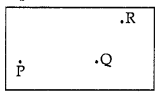Now, to construct a parallelogram with these three non-collinear points P, Q and R, follow the steps given below.Step 1. First, join the points P and Q to make a straight line PQ.
Step 2. Measure PQ with the help of scale. Let PQ be a units.
Step 3. Now, join the points Q and R to make a straight line QR.
Step 4. Measure QR with the help of a scale. Let QR be b units.
Step 5. With P as centre and radius b units, draw an arc of the circle. .
Step 6. With R as centre and radius a units, draw another arc intersecting the arc drawn in step 5.
Step 7. Name the point of intersection of the two arcs as S.
Step 8. Join PS and RS to obtain the desired parallelogram.
Thus, PQRS is the required parallelogram.

Question 3.
ABC is a triangle, obtuse angled at C. How will you construct a perpendicular from B to AC? Construct and show.
Let us first draw a rough figure and then start the construction.Steps of construction:
Step 1. We have given a ∆ABC, obtuse angled at C. First, produce AC to X such that ACX is a straight line.
Step 2. Measure ∠BCX with the help of a protractor. Let it be p°.
Step 3. At B, construct an angle of p° so that ∠CBY = p°.
Step 4. Produce YB to Z so that YBZ is a straight line.
Step 5. Now, line YBZ is parallel to line ACX because ∠BCX = ∠CBY = p°.
Step 6. Now, place tip of the compass on point B and draw an arc of the circle cutting the YZ at points M and N.
Step 7. Without changing the width of the compass (used to draw the arc of the circle in the previous step), place its tip on M and draw an arc cutting the arc drawn in step 5, at point P.
Step 8. Without changing the width of the compass, place its tip on P and draw another arc cutting the arc drawn in step 5 at another point Q.
Step 9. With the tip of the compass still on P, draw another arc somewhere outside.
Step 10. Using the same width of the compass (as set in the previous step), now place its tip on Q and draw another arc which cuts the arc drawn in step 8 at some point (say R).
Step 11. Join the points B and R and extent if to cut the ray AX at W.
Thus, BW is the required perpendicular draw on ray AX.Question 1.
Construct a ∆ABC in which BC = 5 cm, AB = 4 cm, AC = 4.5 cm. Draw the bisectors of ∠B and ∠C to meet at O. Measure ∠BOC.
Steps of Construction: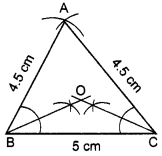1. Draw BC = 5 cm
2. Draw two arcs with centre B and C and radius 4 cm and 4.5 cm respectively to meet A.
3. Join BA and CA.
4. ∆ABC is the required triangle.
5. Draw the bisector of ∠B and ∠C to meet at O.
6. ∠BOC = 130°

Question 2.
Construct a ∆ABC in which BC = 4.8 cm, ∠B = 60°, ∠C = 45 bisectors of AB, BC and CA. Do they pass through the same point?
Steps of Construction: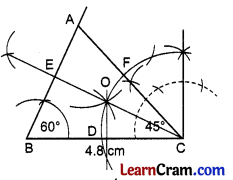1. Draw BC = 4.8 cm
2. Draw ∠B = 60° and ∠C = 45°
3. The two angle lines meet at A.
4. ∆ABC is the required triangle.
5. Draw the perpendicular bisectors of BC, AB and CA at D, E and F respectively to meet at O.

Question 3.
Construct a ∆PQR in which QR = 5 cm, ∠PRQ = 135° and PR = 4 cm. Measure the ∠P and ∠Q.
Steps of Construction: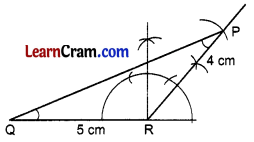1. Draw QR = 5 cm
2. Draw ∠PRQ = 135°.
3. Cut PR = 4 cm.
4. Join PQ.
5. ∆PQR is the required triangle.
6. ∠Q = 20° and ∠P = 25°Question 4.
Construct a right triangle ABC in which BC = 4 cm and hypotenuse makes angle of 45° with BC. Measure AC.
Steps of Construction:1. Draw BC = 4 cm
2. Draw an angle of 45° at C and 90° at B to meet at A.
3. ∆ABC is the required right angle triangle.
4. Measurement of AC = 4 cm.

Question 5.
Construct a ∆ABC in which BC = 4.5 cm, ∠C = 120° and AC = 4.5 cm. Measure ∠A and ∠B.
Steps of Construction: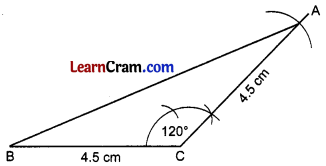1. Draw BC = 4.5 cm
2. Draw ∠C = 120°.
3. Cut CA = 4.5 cm.
4. Join AB.
5. ∆ABC is the required triangle.
6. ∆ABC is an isosceles A in which ∠B and ∠A are 30° each.

Question 6.
Construct a ∆ABC in which BC = 5 cm, ∠B = 120° and ∠C = 80°. Is it possible to construct A ABC? Give reason.
Steps of Construction: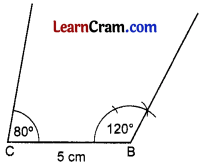1. Draw BC = 5 cm ,
2. Draw ∠B = 120°
3. Draw ∠C = 80°
4. The two angle lines do not cut each other anywhere.
5. Reason:
∠C = 80°, ∠B = 120°
∠C + ∠B = 80° + 120°
= 200° > 180°
6. Hence it is not possible to construct ∆ABC with the given measurement.Question 7.
Draw a ∆ABC in which BC = 5.5 cm, ∠B = 60° and ∠A = 100°.